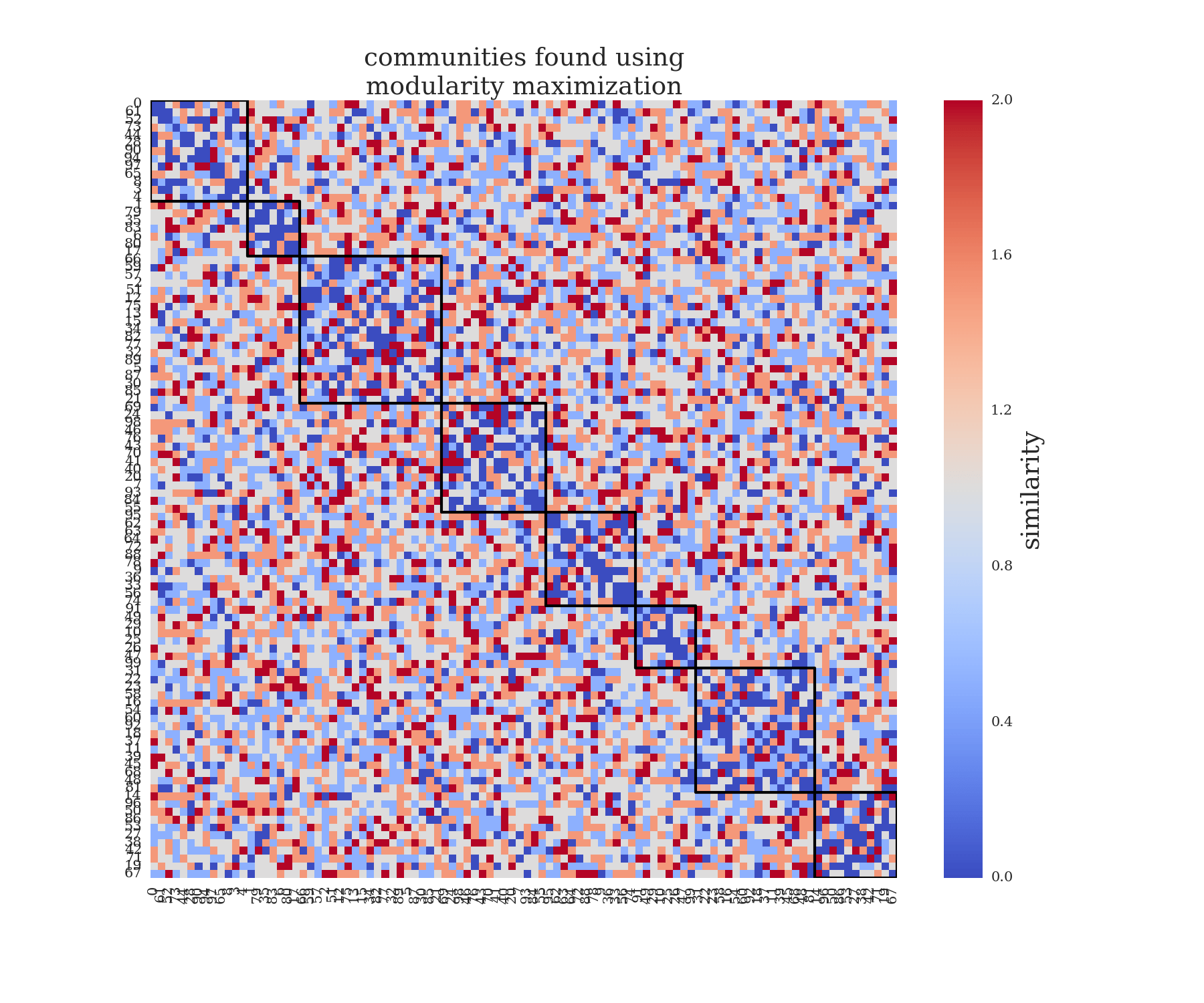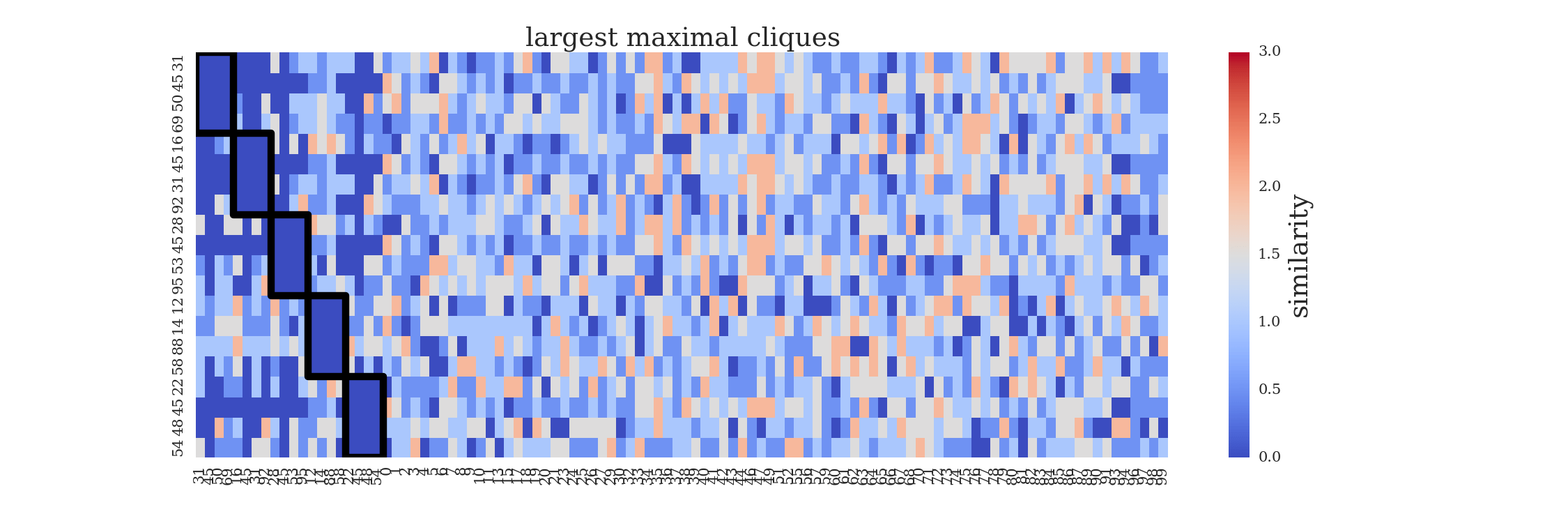## Communities and cliques

Brin Rosenthal (sbrosenthal at ucsd.edu)

You probably won’t get far learning about networks and graph theory before coming across communities and cliques in graphs. At first glance, these two concepts are quite similar- they both describe highly connected sets of nodes, after all. There are however situations which are best suited to one or the other. In this post we will explore some similarities and differences between communities and cliques, and a specific problem I came upon which I thought would be easily solved by a community-finding algorithm, but soon realized that cliques were the much better option!

A network community is a set of nodes which have dense connections within the group, and sparse connections outside the group. A large number of community finding algorithms have been developed, which vary in complexity, and have different strengths and weaknesses depending on context, of which we list a small sampling: Fortunato, S, Malliaros, F, Newman, M., Airoldi, E. et al, Karrer, B., Newman, M., Peixoto, T., Johnson, S.. The community-finding algorithms generally optimize some parameter (usually related to the number of within-group and between-group edges). They are also generally stochastic, meaning that you may get slightly different answers on different runs. It can be useful to explore the community structure of many real-world networks, to establish substructure in the graph. Unlike cliques, there are no exact communities in a graph, rather you will get different answers depending on what algorithm you use, and what you are optimizing for.

A clique is in some sense a stronger version of a community. A set of nodes forms a clique (equivalently, a complete subgraph) if all possible connections between nodes exist. A two-node clique is simply two connected nodes. A three node clique is also known as a triangle. Graphs also contain maximal cliques, which are complete subgraphs such that no other node can be added while maintaining completeness. In Figure 1 below, there are only two maximal cliques of size greater than two. There is a maximal clique of size 5 (orange nodes), and a maximal clique of size 3 (green nodes).Figure 1: Nodes color-coded by clique-membership.

One of the most commonly used maximal clique-finding algorithms may be found here, and recursively searches through the graph finding all maximal cliques. Depending on structure, graphs may contain a large number of cliques, resulting in a high memory cost for the algorithm.

In my research I have mainly focused on applications of community-finding algorithms to biological networks. However, I recently came upon a problem that was solved much better using a clique-finding approach than a community-finding one. Briefly, we had a matrix of similarity between objects (Figure 2), and we wanted to find sets of objects which were all very dissimilar from each other.Figure 2: Randomly generated similarity scores between 100 objects

Application of community-finding algorithms resulted in either groups of highly similar objects grouped together (modularity maximization- Figure 3), or groups of objects which were mostly dissimilar, but contained a couple similar objects in the group. In our application, we couldn’t tolerate any similar objects in our groups, so both of these solutions weren’t satisfactory.Figure 3: Objects clustered by dissimilarity, using modularity maximization algorithm. Groups are only weakly dissimilar, with many similar pairs existing in the same groups.

I then realized that I could reform the problem of finding sets of highly dissimilar objects into a clique-finding framework. I simply created a network from our similarity matrix by connecting nodes whenever they had a similarity level less than a certain tolerance. Once this dissimilarity network was created, it was simply a matter of applying the networkx function find_cliques to the graph. In our network of 100 nodes and 649 edges, there were 362 maximal cliques, with the largest of these maximal cliques containing 4 nodes.

While most community-finding algorithms discretely partition graphs (with some exceptions), nodes can belong to a large number of maximal cliques (see example below- Figure 4). For our problem, this meant we had a large number of maximal cliques to choose from, but many of them contained a lot of the same information.Figure 4: Five largest maximal cliques outlined in black.

We can tune the sizes of our dissimilar sets by changing the threshold of similarity we can tolerate. When we increase the similarity threshold from 0.0 to 1.0, we now have 2840 maximal cliques, and the largest maximal clique contains 6 nodes (Figure 5).Figure 5: Cliques formed with sets of nearly perfectly dissimilar objects.

That’s about all for this post, although please note we have barely scratched the surface of communities and cliques in graphs. Please see the links for more in-depth reading!

« Return